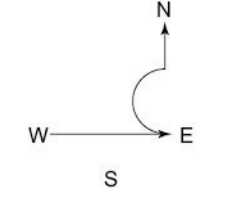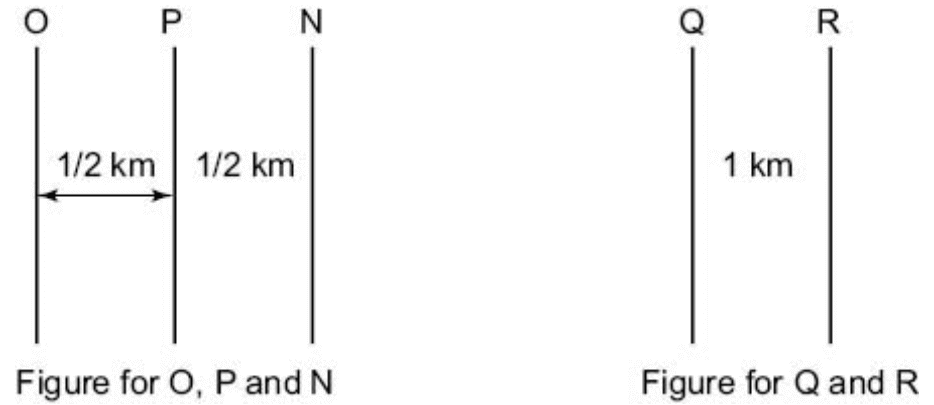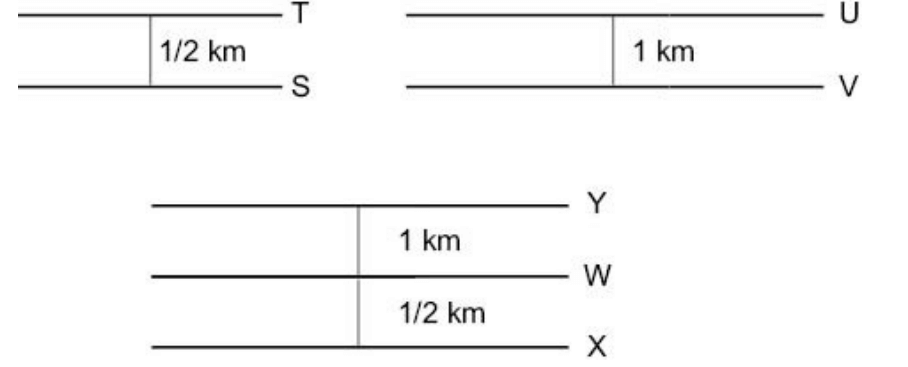## Direction Sense Test Questions and Answers Part-2

1. The Suvarna Rekha river flows from west to east and on the way turns left and goes in a quarter circle around a Shiv temple, and then turns left in right-angles. In which direction is the river finally flowing?
a) North
b) South
c) East
d) West

Explanation:From the given figure it is clear that the river would be flowing north.
Option (a) is correct.

Data for Questions 2 & 4 : j, k, l, m, n, o, p, q and r are nine huts. l is 2 km east of k. j is 1 km north of k and q is 2 km south of j. p is 1 km west of q while m is 3 km east of p and o is 2 km north of p. r is situated just in middle of k and l while n is just in middle of q and m

2. Distance between k and l is:
a) 2 km
b) 1 km
c) 5 km
d) 1.5 km

Explanation: The following figure represents the positioning of the nine huts:Option (a) is correct.

3. Distance between k and r is:
a) 1.41 km
b) 3 km
c) 2 km
d) 1 km

Explanation: Option (c) is correct.

4. Distance between p and q is:
a) 4 km
b) 2 km
c) 1 km
d) 3 km

Explanation: Option (c) is correct.

Data for Questions 5 & 9 : All the streets of a city are either perpendicular or parallel to one another. The streets are all straight. Streets N, O, P, Q and R are parallel to one another. Streets S, T, U, V, W, X and Y are parallel to one another.
(i) Street N is 1 km east of Street O.
(ii) Street O is 1/2 km west of Street P.
(iii) Street Q is 1 km west of Street R.
(iv) Street S is 1/2 km south of Street T.
(v) Street U is 1 km north of Street V.
(vi) Street W is 1/2 km north of Street X.
(vii) Street W is 1 km south of Street Y.

5. If W is parallel to U and W is 1/2 km south of V and 1 km north of T, then which two streets would be 1&1/2 km apart?
a) U and W
b) V and S
c) V and T
d) W and V

Explanation: The vertical North South streets are N, O, P, Q, R. From the basic information we have two relativepositions are available—one between O, P and N and the other between Q and R.The horizontal eastwest streets are: S, T, U, V, W, X, Y.
Of these seven streets the relative positioning is given in 3 distinct parts as shown here.The solution is visible from the figure below. Option (a) is correct6. Which of the following possibilities would make two streets coincide?
a) X is 1/2 km north of U
b) P is 1 km west of Q
c) Q is 1/2 km east of N
d) R is 1/2 km east of O

Explanation: If R is 1/2 km east of O, then R and P would coincide. Option (d) makes two streets coincide

7. If street R is between O and P, then distance between N and Q is:
a) 1/2 KM
b) 1 km
c) 1.5 km
d) 1.5–2 km

Explanation: If R is placed between O and P, Q to N would be a minimum of 1.5 and a maximum of 2 kms. Option (d) is correct

8. If R is between O and P, then which of the following is false?
a) Q is 1.75 km west of N
b) P is less than 1 km from Q
c) R is less than 1 km from N
d) Q is less than 1 km from O

Explanation: If R is between O and P, then Q being 1 km to the west of R, would be more than 1 km to the west of P. Option (b) is correct.

9. Which of the following is necessarily true (given the basic clues)?
a) R and O intersect
b) Q is 2 km west of O
c) Q is at least 2 km west of N
d) Y is 1.5 km north of X